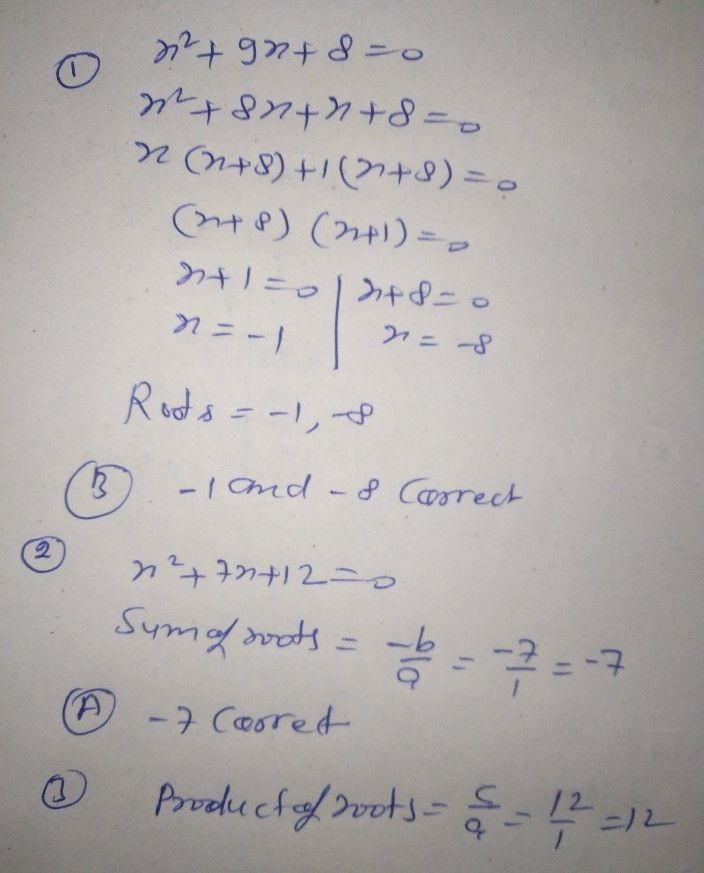Symbol
Problem$8$ What are the roots of the quadratic equation $x^{2}+9x+8=07$ A. 1 and 8 B. $=1$ and $-8$ C. 2 and 4 D. $=2$ and $-4$ $9$ Find the sum of the roots of the quadratic equation $nx^{2}+7x+12=0$ $A$ $=7$ $B.7$ $C.-12$ D. $12$ $10a$ What is the product of the roots of quadratic equation in $n0.97$ A. $-7$ B. 7 $C.-12$ D. $12$
7th-9th grade
Algebra
Search count: 115
SolutionQanda teacher - Lokesh